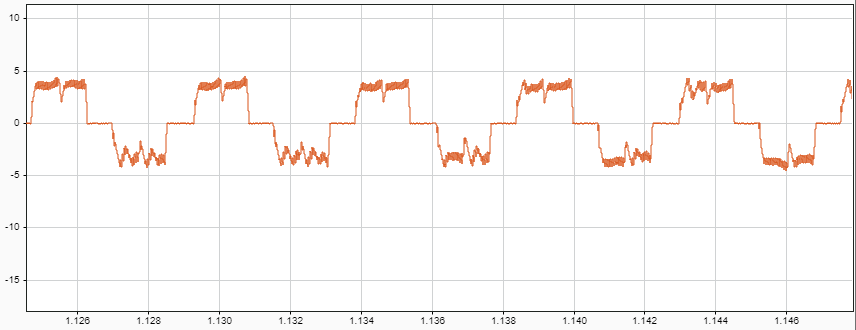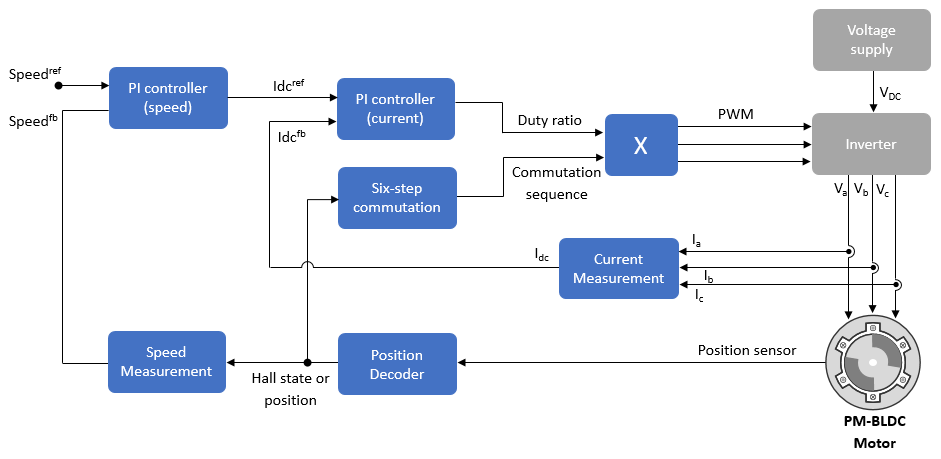## Six-Step Commutation

Six-step commutation, also known as trapezoidal commutation, is a commutation technique used to control three-phase brushless DC (BLDC) permanent magnet motor. It controls the stator currents to achieve a motor speed and direction of rotation.

Six-step commutation uses these conduction modes:

• 120 degree mode conducts current in only two stator phases.

• 180 degree mode conducts current in all three stator phases.

Motor Control Blockset™ supports 120 degree conduction mode. At a given time, this mode energizes only two stator phases and electrically isolates the third phase from the power supply. You can use either Hall or quadrature encoder position sensors to detect the rotor position. Motor Control Blockset provides Six Step Commutation block that uses the Hall sequence or rotor position inputs to determine the 60 degree sector where the rotor is present. It generates a switching sequence that energizes the corresponding phases. As the motor rotates, the sequence switches the stator currents every 60 degree such that the torque angle (angle between rotor d-axis and stator magnetic field) remains 90 degrees (with a deviation of 30 degrees). Therefore, the switching signals operate switches to control the stator currents, and therefore, control the motor speed and direction of rotation. For more details, see Six Step Commutation.

The stator current waveform takes a trapezoidal shape.The 120 degree conduction mode is a less complex technique that provides good speed control for the BLDC motors. This figure shows the six-step commutation architecture for a BLDC motor.Get ebook